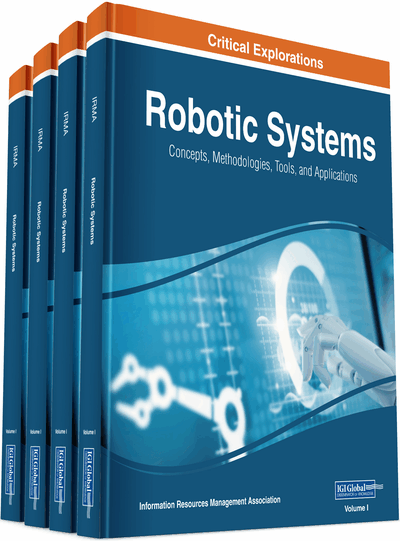# Multi-Robot Swarm for Cooperative Scalar Field Mapping

Hung Manh La
DOI: 10.4018/978-1-7998-1754-3.ch010
OnDemand:
(Individual Chapters)
Available
\$37.50
No Current Special Offers

## Abstract

In this chapter, autonomous mobile robots are deployed to measure an unknown scalar field and build its map. The development of a cooperative sensing and control method is presented for multi-robot swarming to build the scalar field map. The proposed method consists of two parts. First, the development of a distributed sensor fusion algorithm is obtained by integrating two different distributed consensus filters to achieve cooperative sensing among robots. This fusion algorithm has two phases. In the first phase, the weighted average consensus filter is developed which allows each robot to find an estimate of the value of the scalar field. In the second phase, the average consensus filter is used to allow each robot to find a confidence of the estimate. The final estimate of the value of the scalar field is iteratively updated during the movement of the robot via a weighted average protocol. Second, the distributed control algorithm is developed to control the mobile robots to form a network and cover the field. Experimental results are provided to demonstrate the proposed algorithms.
Chapter Preview
Top

## System Modeling

In this section we present the models of multi-robot system, scalar field, and sensor measurement of each robot.

### Multi-Robot System Model

Consider a dynamic graph G consisting of a vertex set ϑ = {1, 2..., n} and an edge set E⊆ {(i, j): i, j ϑ, j≠ i}. In this graph each vertex denotes a mobile robot, and each edge denotes the communication link between robots.

Letbe the position of robot i, and dij(t) = ||pjpi|| be the Euclidean distance. During the movement of the robots, the relative distance between them may change, hence the neighbors of robot also change. Therefore, a neighborhood set of robot i at time step t can be defined as:

(1) where r is a robot sensing range.

Consider n mobile robots moving in a two dimensional space and each robot with its dynamic as:

(2) whereis the velocity vectors of robot i, respectively. The control input of robot i is

(3)

## Complete Chapter List

Search this Book:
Reset